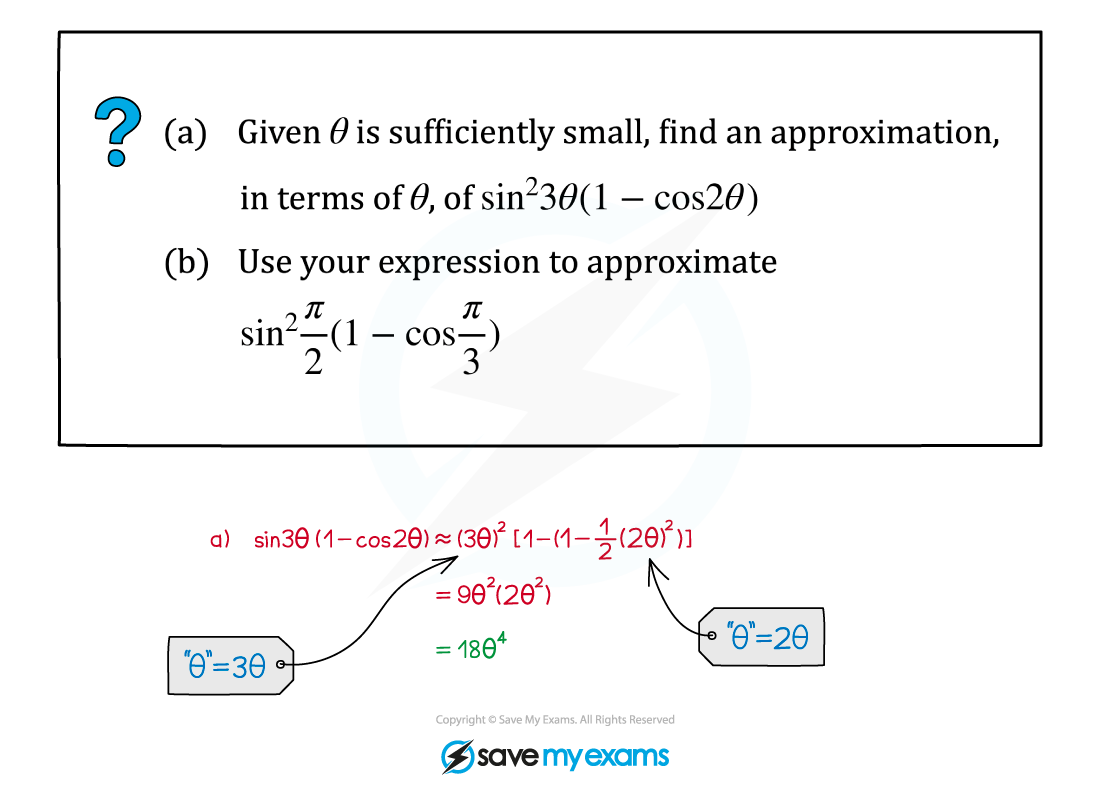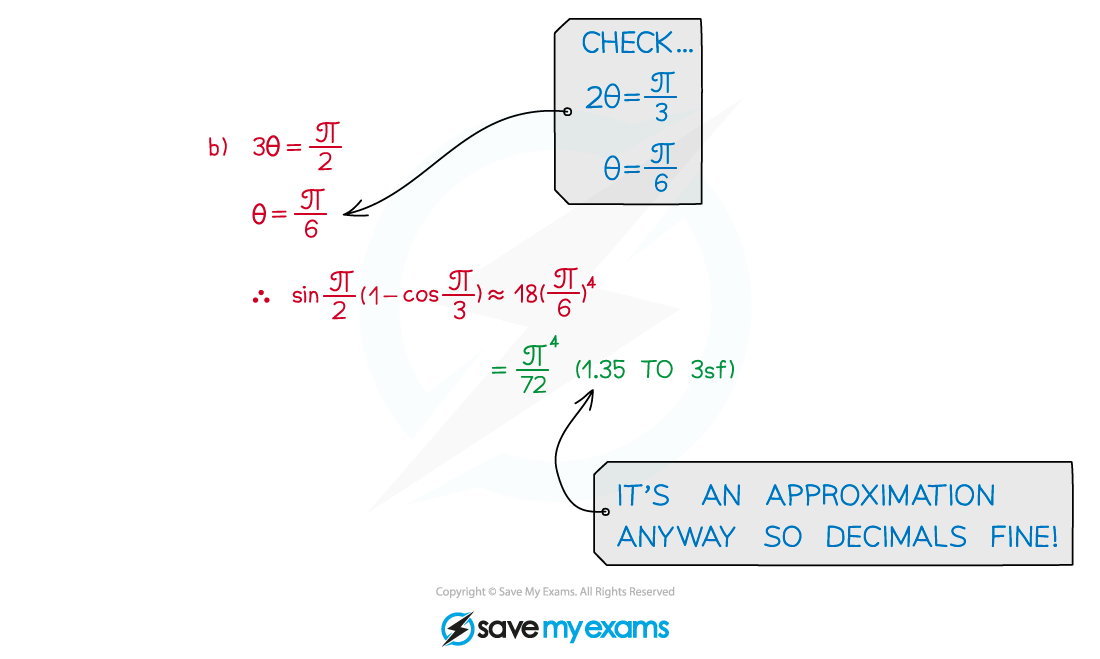# AQA A Level Maths: Pure复习笔记5.4.3 Small Angle Approximations

### Small Angle Approximations

#### Small angle approximations

• When an angle measured in radians is very small, you can approximate the value using small angle approximations
• These only apply when angles are measured in radians
• They can be applied to positive and negative small angles

#### What's the small-angle approximation of sin θ?

sin θ ≈ θ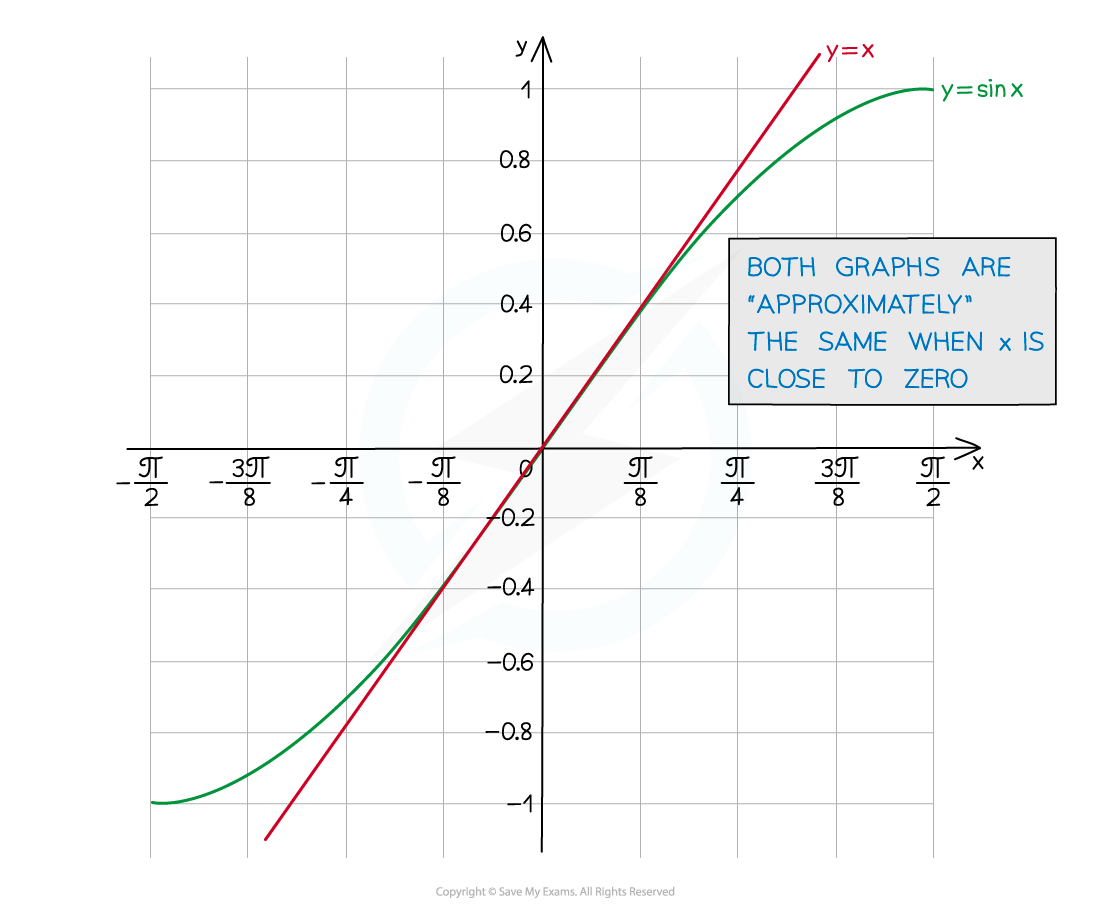#### What's the small-angle approximation of cos θ?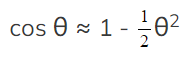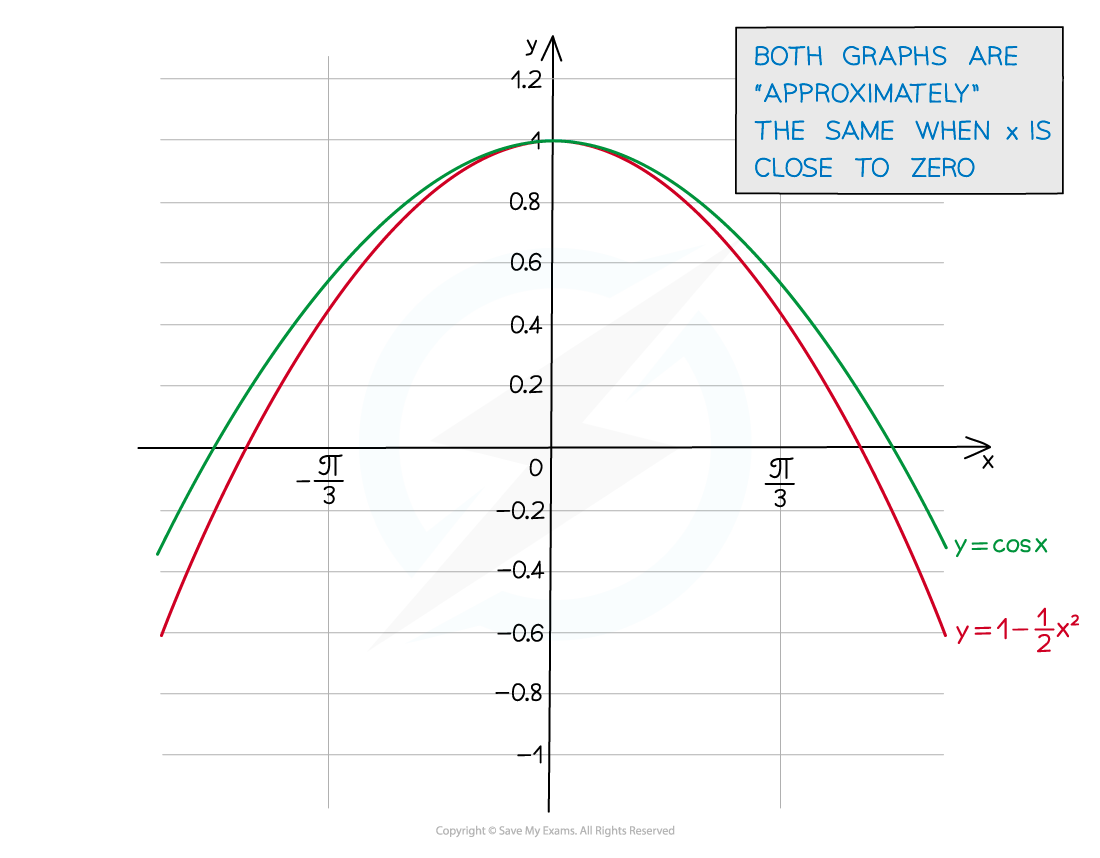• y = cos θ (near zero) is similar to a “negative quadratic” (parabola)

#### What's the small-angle approximation of tan θ?

tan θ ≈ θ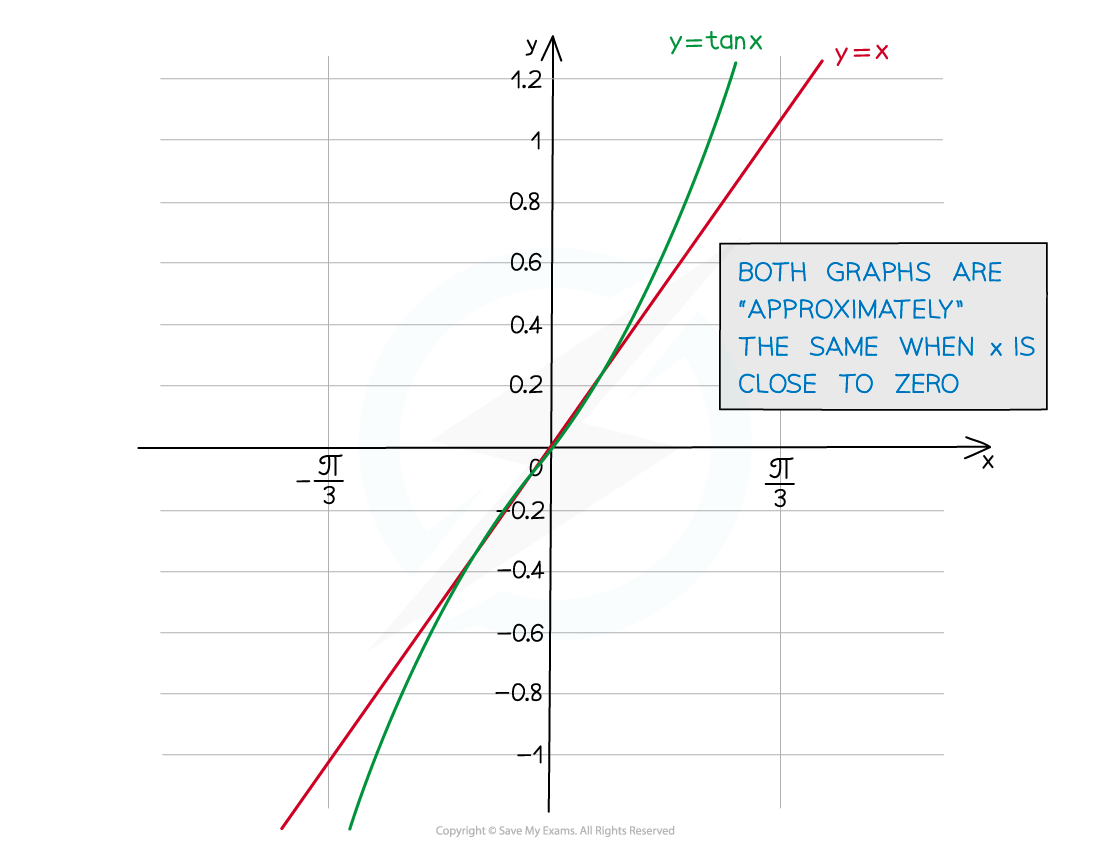#### How do I use small angle approximations in solving problems?

• Replace sin θ, cos θ or tan θ with the appropriate approximation
• Given angles are often 2θ, 3θ, …
• Replace “θ” in the approximation by 2θ, 3θ, …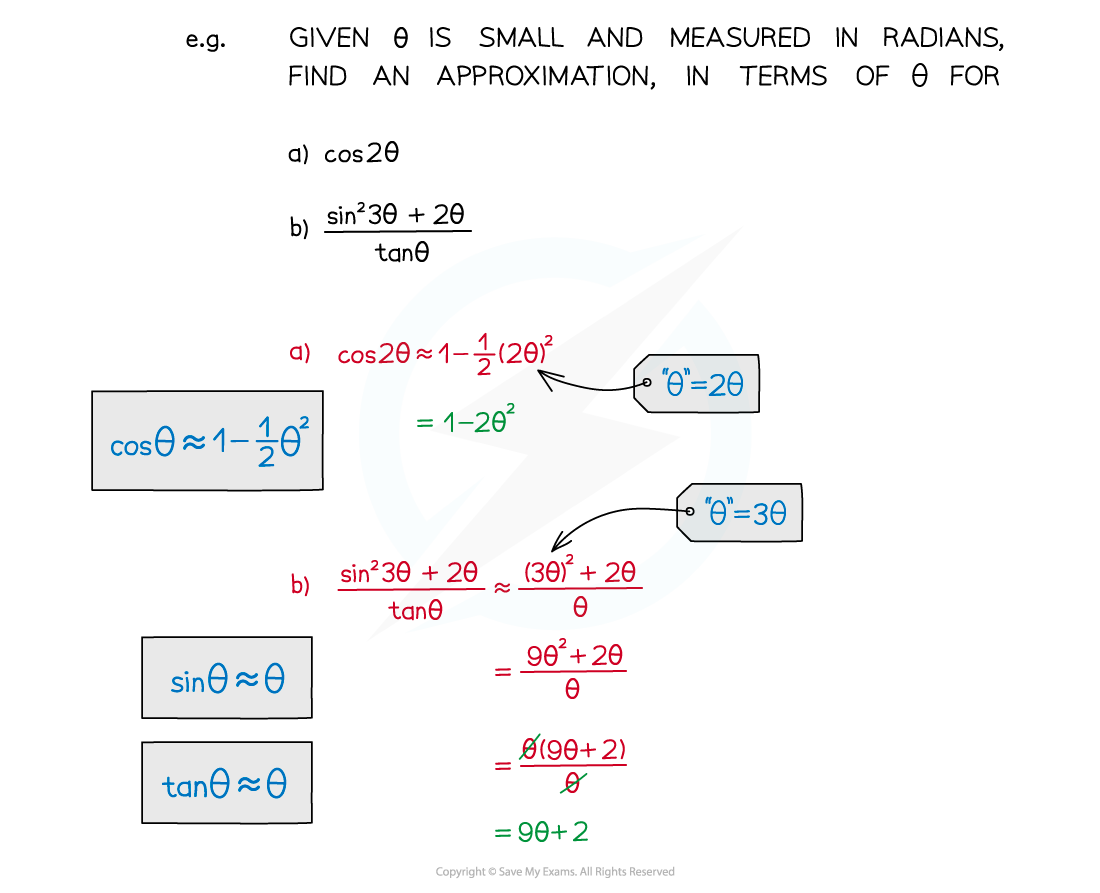• Binomial expansion (see GBE) may be involved in more awkward expressions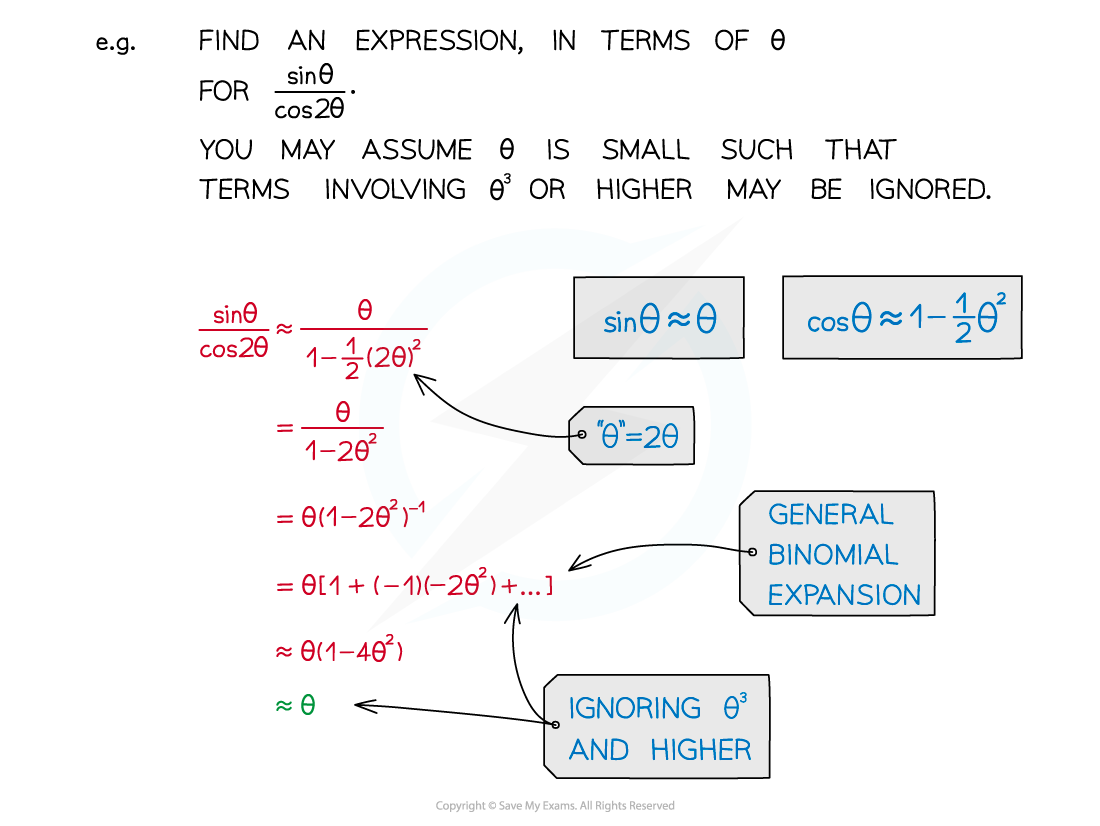#### Exam Tip

• Small angle approximations are given in the formula booklet.
• They can be used in proofs – particularly differentiation from first principles (see First Principles Differentiation - Trigonometry).

#### Worked Example